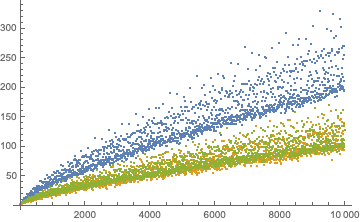top of page
All Posts: Blog2

# [Research] Goldbach's Comet, frequency graph, and a little bit on time complexity

Updated: Dec 15, 2020

Goldbach's conjecture, one of the simplest yet most difficult problem in number theory, claims that

Goldbach's Conjecture

Any positive even integer greater than 4 can be expressed as the sum of two odd primes.

This seemingly straightforward problem has, surprisingly, confused thousands of mathematicians and intellectuals. But although it is impossible to determine whether the conjecture is true or not in my level, we can receive the aid of computers to discover important properties of mathematics.

All even integers that a computer could calculate have been found to be the sum of two odd primes. However, there are some numbers that have more couples of such two odd primes. For instance, 22 can be expressed as the sum of (3,19), (5,17), and (11,11)-- all three couples in total. But is there a pattern on how many couples are possible for each even number?

For even numbers from 2 to 10,000, we could obtain Fig 1.1, where a set of one thick line, a thinner line, and other lines are slightly visible as well. Expanding onto 100,000, we could obtain Fig 1.2, where the lines and spaces became much starker and visible.Fig 1.1. Number of prime couples that sum to even numbers from 2 to 10,000Fig 1.2. Number of prime couples that sum to even numbers from 2 to 100,000

I am not entirely clear about why this is happening, but putting the dots under mod 6, I was able to get the shape as Fig 2. The blue dots are the number of prime couples that sum up to an integer divisible by 6. The green and orange dots are for integers that are 2 and 4 under modulo 6, respectively.Fig 2. Prime couples that sum up to integers that have

a remainder of 0, 2, 4 under modulo 6, respectively

My intuition was that the difference between consecutive primes might offer some insight. However, this effort ended in futile results, so my next idea was to represent the number of prime couples that sum to n (I will denote this as G(n) afterwards) as some closed form. However, if that was possible, Goldbach's conjecture would've been already proved. My final try was to first plot all prime numbers, which would produce one line. Then, by summing each of the prime numbers one by one, it would create more and more lines. But this was also unsuccessful, as it was impossible to prove why this shape was being produced, and explain why some regions were left vacant.

Now that all of my efforts ended up unsuccessfully, I went online to search about the cause, and I found that this shape is actually called Goldbach's Comet, which I came across while searching why this is happening. G. H. Hardy actually wrote a proof on why this is happening. Look at pages 1-70 in the link below.

An additional investigation I constructed is how many even numbers have a value of each of the values for G(n). That is, only 10 even numbers have a value of G(n)=1, and 17 have G(n)=2. On the x axis of the diagram below is each of the values for G(n), and on the y-axis is the numbers of n that give each value of G(n). For both Fig 3.1 and Fig 3.2, there is a general trend of a rapid increase followed by a rapid decrease, a slight rebounding, and a slow decrease again. But this decreasing trend seems to happen because we stopped computing for n larger than a certain limit. If n was set at infinity, the graph would constantly increase; hence, this shape might not be a product of some interesting property, but a product of the methodology itself.Fig 3.1. number of n for each G(n) for n=2 to 10,000Fig 3.2. number of n for each G(n) for n=2 to 100,000

Just as a sidekick to this investigation, it was also quite interesting how I would code for these massive numbers to minimize time complexity. From 100,000 onwards, my laptop was unable to do the computing with the original code, so I had to devise a new code as the below. For both codes, the input is to which number we will compute G(H). Note that the second code is much simpler, as it has to only compute pC2 times plus a few more p times.

Original Code to get Goldbach's Comet:

```primeFunction[i_] := Module[{},
primeTable[i] = Table[PrimeQ[j], {j, 1, i}];
allPrimes[i] = Pick[Table[j, {j, 1, i}], primeTable[i]];
nPrimes[i] = Length[allPrimes[i]];
primeData[i] = Table[{j, allPrimes[i][[j]]}, {j, 1, nPrimes[i]}]]```

New Code for Goldbach's Comet:

```primeFunction[i_] := Module[{},
allPrimes = Most[NestWhileList[NextPrime[#] &, 3, # < i &]];
allPairs = Subsets[allPrimes, {2}];
pairSums = Plus @@@ allPairs;
tally = Tally[pairSums];
Select[tally, #[] <= i &]]```

Code for colour coded comet:

```data6 = Table[{6 i, primeFunction[6 i]}, {i, 1, 1666}];
data62 = Table[{6 i + 2, primeFunction[6 i + 2]}, {i, 0, 1666}];
data64 = Table[{6 i + 4, primeFunction[6 i + 4]}, {i, 1, 1666}];
ListPlot[{data6, data62, data64}]```

Code for computing number of n for each value of G(n):

```dataNum = Table[primeData[[i]][], {i, 1, 49997}];
maxSum = primeData[[Ordering[dataNum, -1]]][][]
dataSum = Table[Count[dataNum, i], {i, 1, maxSum}];
ListPlot[dataSum, ImageSize -> Large]```
37 views

See All
bottom of page